Скачать презентацию Non-Inertial Frames of Reference. Forces of Inertia

non-inertial_frames_forces_of_inertia.ppt

• Размер: 195 Кб
• Количество слайдов: 17

## Описание презентации Non-Inertial Frames of Reference. Forces of Inertia по слайдамNon-Inertial Frames of Reference. Forces of Inertia • Non-Inertial Frames of Reference • Forces of Inertia • Principle of Equivalence of Force of Gravity and Force of Inertia • Forces of Inertia in Rotating Frame of Reference • Centrifugal Force • Coriolis ForceNon-inertial Frames of Reference. Forces of Inertia • Non-Inertial Frames of Reference • Forces of Inertia • Principle of Equivalence of Force of Gravity and Force of Inertia • Forces of Inertia in Rotating Frame of Reference • Centrifugal Force • Coriolis ForceNon-inertial Frames of Reference The frame is called non-inertial one if it moves with acceleration relative to an inertial frame of reference.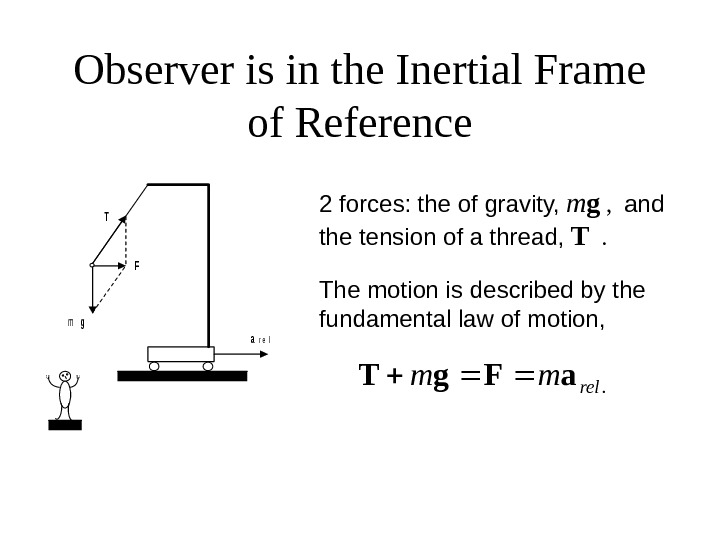Observer is in the Inertial Frame of Referencemg F T ar e l 2 forces: the of gravity, m g , and the tension of a thread, T . The motion is described by the fundamental law of motion, . relmma. Fg. TObserver is in the Non-Inertial Frame of Referencemg T ar e l The ball is in state of rest! Newton’s law of motion seems to be not correct now!?Non-Inertial Frames of Reference. Forces of Inertia • Non-Inertial Frames of Reference • Forces of Inertia • Principle of Equivalence of Force of Gravity and Force of Inertia • Forces of Inertia in Rotating Frame of Reference • Centrifugal Force • Coriolis Force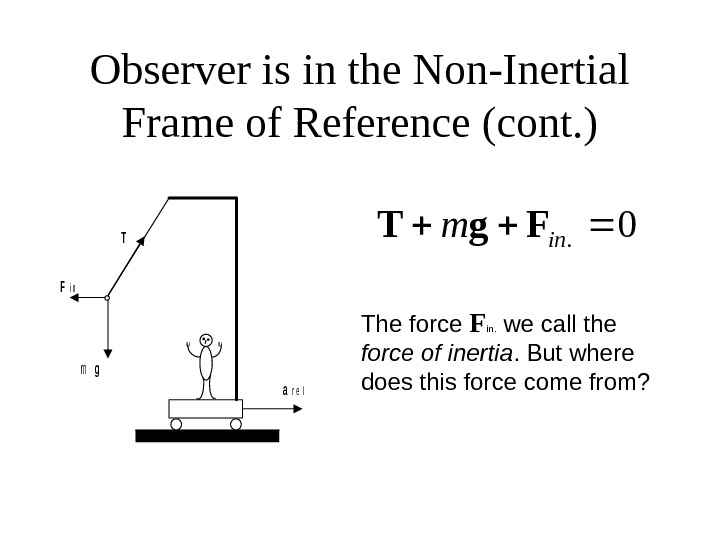Observer is in the Non-Inertial Frame of Reference (cont. )mg Fi n T ar e l 0. inm. Fg. T The force F in. we call the force of inertia. But where does this force come from?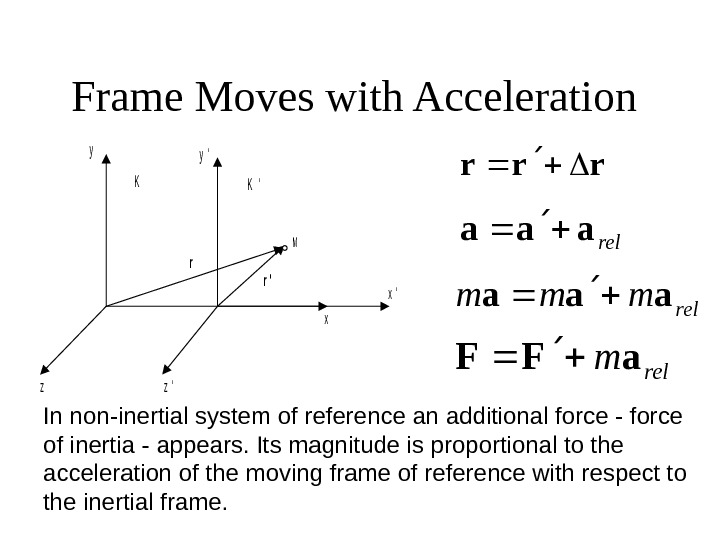Frame Moves with Acceleration yy ‘ KK ‘ M r r ‘ x x ‘ zz ‘ rrr relaaa rel mmm aaa relma. FF In non-inertial system of reference an additional force — force of inertia — appears. Its magnitude is proportional to the acceleration of the moving frame of reference with respect to the inertial frame.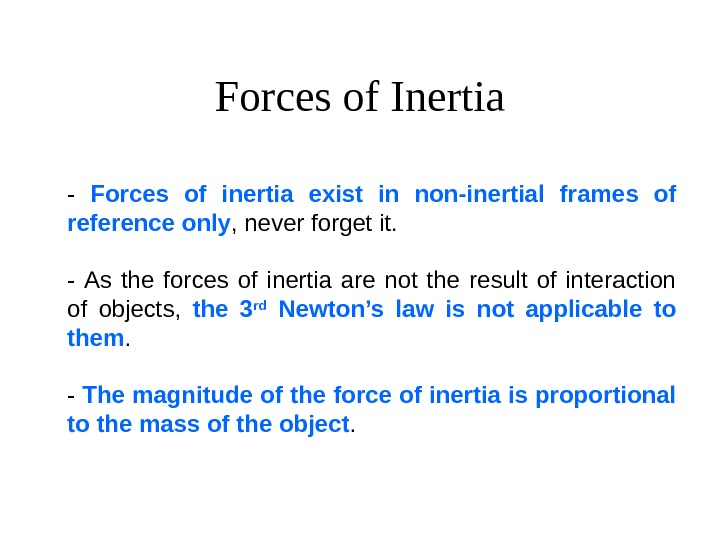Forces of Inertia — Forces of inertia exist in non-inertial frames of reference only , never forget it. — As the forces of inertia are not the result of interaction of objects, the 3 rd Newton’s law is not applicable to them. — The magnitude of the force of inertia is proportional to the mass of the object.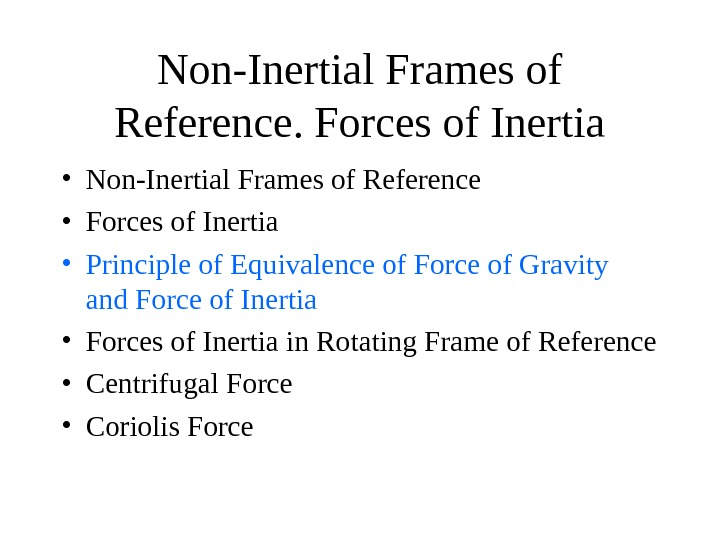Non-Inertial Frames of Reference. Forces of Inertia • Non-Inertial Frames of Reference • Forces of Inertia • Principle of Equivalence of Force of Gravity and Force of Inertia • Forces of Inertia in Rotating Frame of Reference • Centrifugal Force • Coriolis Force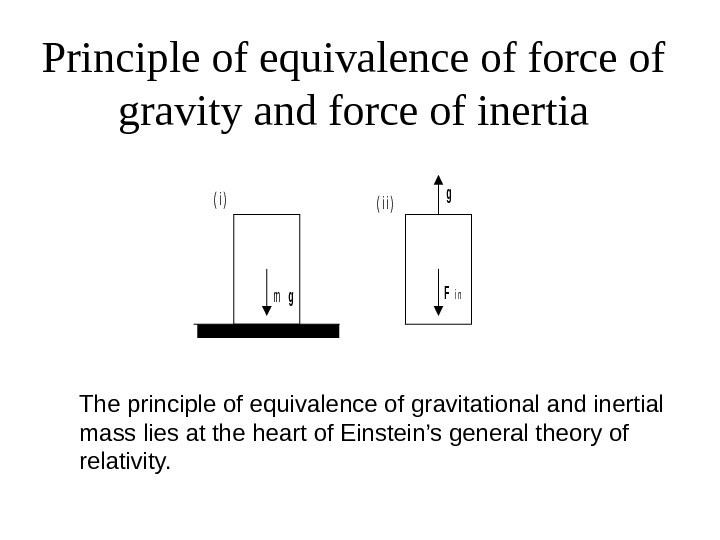Principle of equivalence of force of gravity and force of inertiamg g Fi n ( i )( i i ) The principle of equivalence of gravitational and inertial mass lies at the heart of Einstein’s general theory of relativity.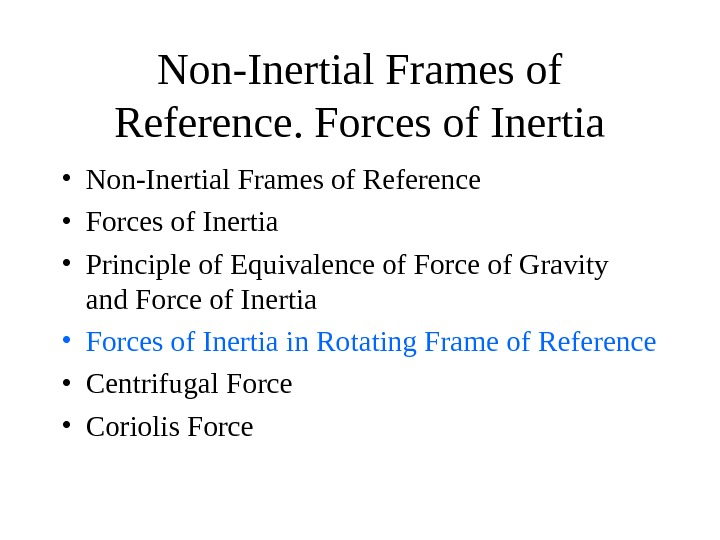Non-Inertial Frames of Reference. Forces of Inertia • Non-Inertial Frames of Reference • Forces of Inertia • Principle of Equivalence of Force of Gravity and Force of Inertia • Forces of Inertia in Rotating Frame of Reference • Centrifugal Force • Coriolis ForceForces of Inertia in Rotating Frame of Reference K K ‘ v ‘ RO In frame the motion of the ball is uniform circular motion with . In K frame the motion of the ball is uniform circular motion, its velocity is . K constv Rvv The centripetal acceleration of the ball in K is nnnnna 2 v. R Rv Rv cp 2)()( 222 nnaavm. Rmmmcpcp 2 2 Multiplying by mass of the ball,Centrifugal and Coriolis Forcesnn. Favm. Rmmcp 2 2 centrifugal force of Coriolis RF 2 mcf]], [[RωωFmcf ], [2ωv. Fmcor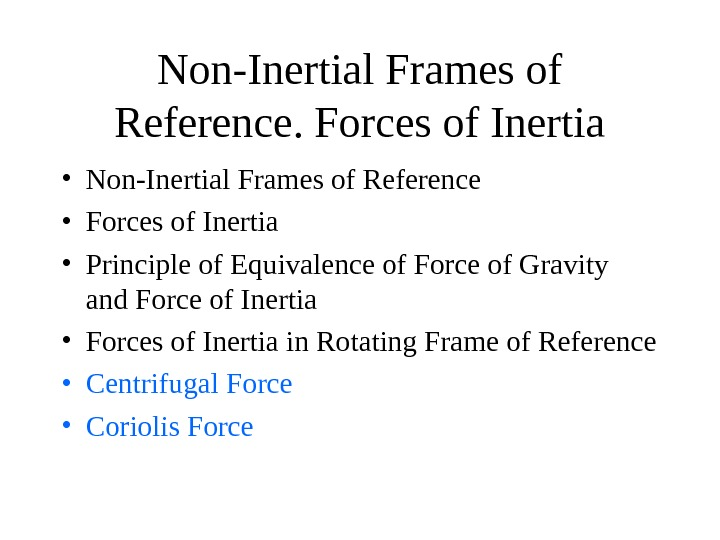Non-Inertial Frames of Reference. Forces of Inertia • Non-Inertial Frames of Reference • Forces of Inertia • Principle of Equivalence of Force of Gravity and Force of Inertia • Forces of Inertia in Rotating Frame of Reference • Centrifugal Force • Coriolis Force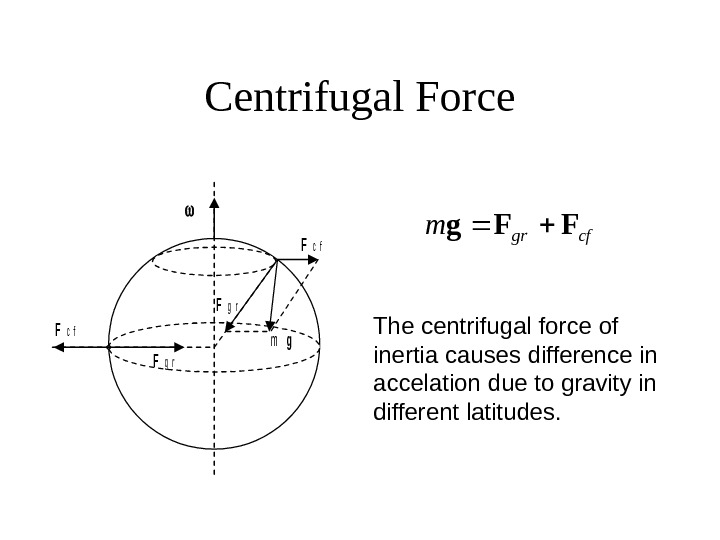Centrifugal Force Fc f Fg r Fc f mg cfgrm. FFg The centrifugal force of inertia causes difference in accelation due to gravity in different latitudes.Coriolis Force The rivers in the northern hemisphere destroy their right banks. A trajectory of a free falling body deviates to the east. v ‘Fc o r